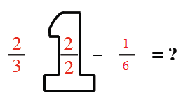### Home > MC2 > Chapter 2 > Lesson 2.1.1 > Problem2-9

2-9.

Simplify the following expressions.

1. $\frac { 2 } { 3 } - \frac { 1 } { 6 }$

Convert fractions to like terms with common denominators before subtracting.Try solving after filling in the giant one and converting the fraction.

$\frac{3}{6} \text{ or } \frac{1}{2}$
Now, attempt part (b) and (c) using the same strategy.

1. $\frac { 7 } { 10 } - \frac { 1 } { 5 }$

1. $\frac { 1 } { 2 } - \frac { 3 } { 4 }$